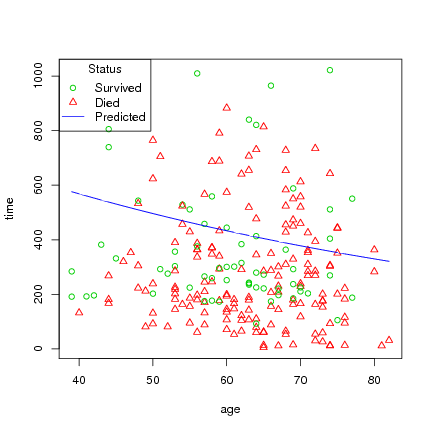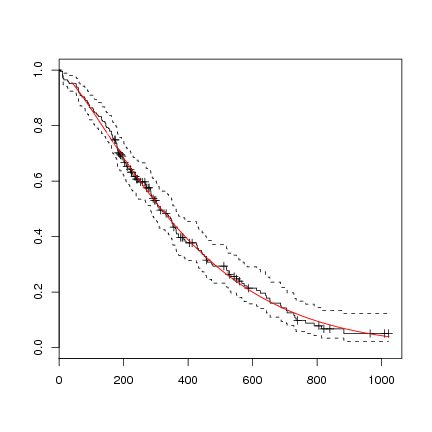DATA MINING
Desktop Survival Guide
by Graham Williams### Lung

 ```> l.survreg <- survreg(l.Surv ~ age, data=lung) > summary(l.survreg) ```

 ```Call: survreg(formula = l.Surv ~ age, data = lung) Value Std. Error z p (Intercept) 6.8871 0.4466 15.42 1.20e-53 age -0.0136 0.0070 -1.94 5.19e-02 Log(scale) -0.2761 0.0624 -4.43 9.61e-06 Scale= 0.759 Weibull distribution Loglik(model)= -1151.9 Loglik(intercept only)= -1153.9 Chisq= 3.91 on 1 degrees of freedom, p= 0.048 Number of Newton-Raphson Iterations: 5 n= 228 ```

 ```> l.pred <- predict(l.survreg, lung) > l.pred.q <- predict(l.survreg, lung, type="quantile") > result <- cbind(data.frame(lung\$time, l.pred), l.pred.q) > names(result) <- c("Actual", "Predicted", "Lower", "Upper") > head(result) ```

 ``` Actual Predicted Lower Upper 1 306 357.8476 64.88637 673.7982 2 455 388.2918 70.40663 731.1220 3 1010 457.1705 82.89600 860.8152 4 210 450.9914 81.77557 849.1803 5 883 432.9505 78.50432 815.2108 6 1022 357.8476 64.88637 673.7982 ```

Plot age versus time, and status, then draw line byt age with the predicted survival time:

 ```> ord <-order(lung\$age) > age_ord <- lung\$age[ord] > pred_ord <- l.pred[ord] > with(lung, plot(age, time, pch=status, col=4-status)) > lines(age_ord, pred_ord, col=4) > legend("topleft", title="Status", c("Survived", "Died", "Predicted"), pch=c(1, 2, -1), lty=c(0,0,1), col=c(3,2,4)) ```You can not plot the survreg directly, but could do the following, using the coefficients from the regression formula, which will also give a hint in interpreting the formula:

 ```> l.survreg.weibull <- survreg(l.Surv ~ 1, data=lung, dist='weibull') > plot(survfit(l.Surv~1, data=lung)) > curve(exp(-(exp(-l.survreg.weibull\$coef) * x)^(1/l.survreg.weibull\$scale)), col="red", add=TRUE) ```Another example this time we fit a parametric survival model with a Weibull distribution for time to death fitting a different shape parameter for each gender, by using a strata term.

 ```> l.survreg <- survreg(l.Surv ~ ph.ecog + age + strata(sex), lung) > print(l.survreg) ```

 ```Call: survreg(formula = l.Surv ~ ph.ecog + age + strata(sex), data = lung) Coefficients: (Intercept) ph.ecog age 6.73234505 -0.32443043 -0.00580889 Scale: sex=1 sex=2 0.7834211 0.6547830 Loglik(model)= -1137.3 Loglik(intercept only)= -1146.2 Chisq= 17.8 on 2 degrees of freedom, p= 0.00014 n=227 (1 observation deleted due to missingness) ```

 ```> summary(l.survreg) ```

 ```Call: survreg(formula = l.Surv ~ ph.ecog + age + strata(sex), data = lung) Value Std. Error z p (Intercept) 6.73235 0.42396 15.880 8.75e-57 ph.ecog -0.32443 0.08649 -3.751 1.76e-04 age -0.00581 0.00693 -0.838 4.02e-01 sex=1 -0.24408 0.07920 -3.082 2.06e-03 sex=2 -0.42345 0.10669 -3.969 7.22e-05 Scale: sex=1 sex=2 0.783 0.655 Weibull distribution Loglik(model)= -1137.3 Loglik(intercept only)= -1146.2 Chisq= 17.8 on 2 degrees of freedom, p= 0.00014 Number of Newton-Raphson Iterations: 5 n=227 (1 observation deleted due to missingness) ```